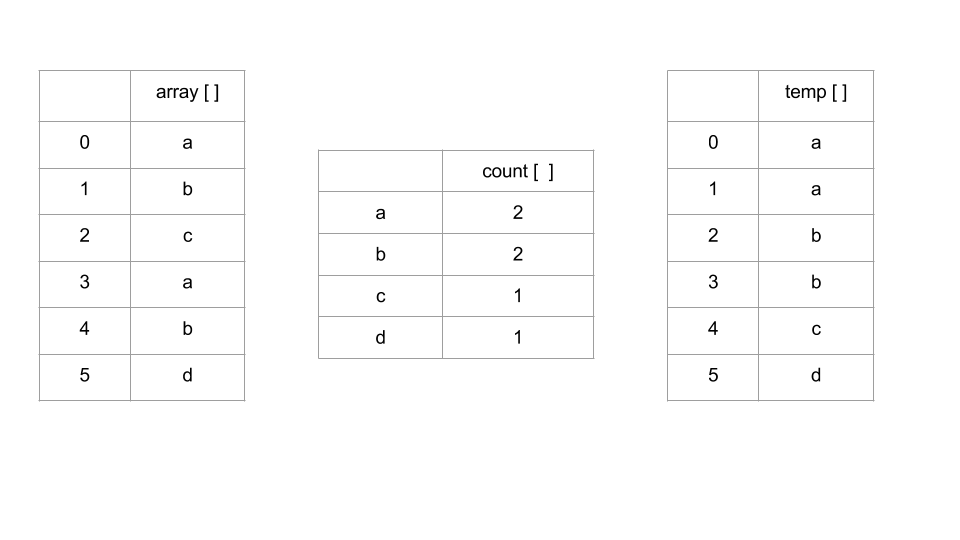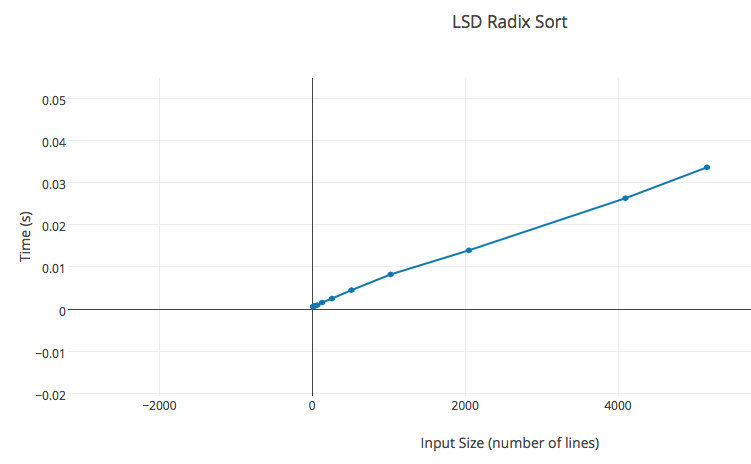# Random Wits

Life is too short for a diary

## Random

\$ random stuff     ├── tags     ├── bookshelf     ├── resources     ├── quotes     ├── habits     └── about me
+ say Hello

Fri 20 Nov 2015

### Quickly sort large file in linear time

Any deterministic general sorting algorithm has average case time complexity $\Omega(n\log{}n)$ . However, certain sorting algorithm can run faster in $O(n)$ but with limitation1. Instead of comparision-based sort, each element is looked individually by its value. Radix sort is one fine example of integer sorting.# Problem Statement

Suppose, we have large directory containing names of people in random order. The names need not be distinct. We need to sort the name in the ascending order. Also our algorithm should run in linear time. A sample of the input file is shown below2

JAMES          1.664  1.664      1
TUSHARSHARMATUSHARSHARMA   1.99 3
JOHN           1.642  3.305      2
ROBERT         1.576  4.881      3
MICHAEL        1.321  6.202      4
MARY           1.319  7.521      5
WILLIAM        1.230  8.750      6
DAVID          1.185  9.934      7
RICHARD        0.854 10.788      8
CHARLES        0.765 11.552      9
JOSEPH         0.705 12.257     10
THOMAS         0.692 12.948     11
PATRICIA       0.539 13.486     12
CHRISTOPHER    0.519 14.005     13
LINDA          0.518 14.523     14
BARBARA        0.490 15.013     15


# Desired Output

The output file contains the names read from the file and sort them alphabetically.

AARON
ABBEY
ABBIE
ABBY
ABDUL
ABE
ABEL
ABIGAIL
ABRAHAM
ABRAM


NOTE: Each line is read upto k = 21 characters (arbitrary). Any character after 21st position is truncated.

Radix sorts the integer by their least significant $\mathtt{d}$ bits. $\mathtt{w}/\mathtt{d}$ passes are made to counting-sort which sorts $\mathtt{w}$-bit integers. Following could be easily implemented in C++.

void lsdRadixSort(char S[][k])
{

for (int d = k - 1; d >=0 ; d--) {
countingSort(S, d);
}

}


Procedure lsdRadixSort reads an array S of size n * k. For each d integers, it passes array and the index to countingSort procedure. The main sorting occurs at Counting Sort.

# Counting Sort

Counting sort an array of integer of length n. Radix sort uses several passes of Counting Sort to sort the array of size n * k . The algorithm works like this

1. Count frequencies of each letter using key as index

2. Compute frequency cumulates

3. Access cumulates using key as index to find record positions.

4. Copy back into original arrayFollowing is the C++ implementation of Counting Sort.

void countingSortSwap(char S[][k], int j)
{
//here j is the column

int count = {0};

char temp[n][k];
temp = ' ';

for (int i = 0; i < n; i++) {
int valueChar = (int) S[i][j];
count[valueChar + 1]++;
}

for (int p = 1; p < 256; p++) {
count[p] += count[p - 1];
}

for (int i = 0; i < n; i++) {
int valueChar = (int)S[i][j];
int index = count[valueChar++]++;
for (int p = 0; p < k; p++) {
temp[index][p] = S[i][p];
}
}

for (int i = 0; i < n; i++) {
for (int p = 0; p < k; p++) {
S[i][p] = temp[i][p];
}
}

}


# More Efficiently?

Radix sort can also be implemented without avoiding the hassle of moving the array to and fro. We use a pointer array (array of string indices) indexP[0..n−1]. Initially, set indexP[i] = i for all i. During the sorting, we move these indices. At the end of sorting, indexP will be the index of the string which is the first in the sorted order, indexP the second string in the sorted order, and so on.

We also declare prevIndex[0..n-1] to keep track of indicies of previous sorting. Thus our lsdRadixSort procedure would be slighlty different as

void lsdRadixSort(char S[][k], int indexP[])
{
int count[k];
int prevIndex[n];

for (int d = 0; d < n ; d++ )
prevIndex[d] = d;

for (int d = k - 1; d >=0 ; d--) {
countingSortp(S, d, indexP, prevIndex);
}

}


Following is the new C++ implementation of Counting Sort.

void countingSort(char S[][k], int j, int indexP[], int prevIndex[])
{
//here j is the column

int count = {0};

int tempCount[n];
memset(tempCount, 0, sizeof(int) * n);

for (int i = 0; i < n; i++) {
int valueChar = (int) S[prevIndex[i]][j];
count[valueChar + 1]++;
}

for (int p = 1; p < 256; p++) {
count[p] += count[p - 1];
}

for (int i = 0; i < n; i++) {
int valueChar = (int)S[prevIndex[i]][j];
int index = count[valueChar++]++;

tempCount[index] =   prevIndex[i];
}

for (int i = 0; i < n; i++) {
indexP[i] = tempCount[i];
prevIndex[i] = tempCount[i];
}
}



Finally we print the names in ascending order in the file as per as the sorted indexP[0 .. n-1].

printToFile(char S[][k], string filename, int indexP[])
{
int tempP[n];
memset(tempP, 0, sizeof(int) * n);

fo.open(filename.c_str(), ios::out | ios::binary);
if (!fo) {
cout<<"Error opening the file\n";
exit(-1);
}
for (int i = 0; i < n; i++) {
for (int j = 0; j < k; j++) {
fo <<S[indexP[i]][j];
}
fo<<endl;
}

}


# Conclusion

The sort runs in linear time as discussed earlier. Below is time it took against input of different size.

 n k time (s) 8 21 0.000591 16 21 0.000674 32 21 0.000762 64 21 0.000961 128 21 0.001582 256 21 0.002517 512 21 0.004514 1024 21 0.008236 2048 21 0.013947 4096 21 0.0263 5164 21 0.033631